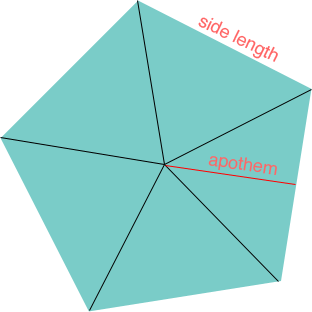SEARCH HOMEMath Central Quandaries & QueriesQuestion from Jenna, a student: I would like to know if there is a formula to find the area of a regular Pentagon that is not inscribed in a circle only apothem and side length are given. Thank youHi Jenna,

If you construct lines from each vertex to the centre you divide the pentagon into 5 congruent triangles.The area of the pentagon is 5 times the area of each triangle and the area of each triangle is

1/2 × base × height = 1/2 × (side length) ×apothem.

PennyMath Central is supported by the University of Regina and The Pacific Institute for the Mathematical Sciences.